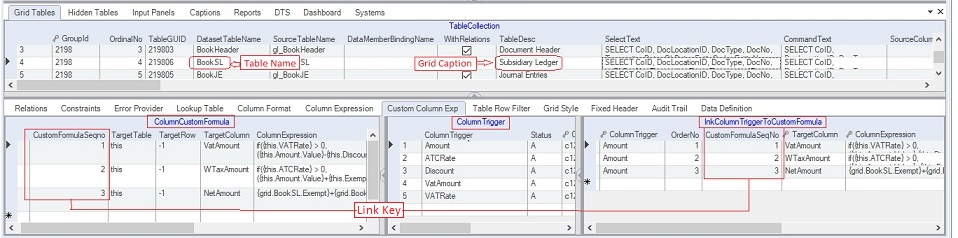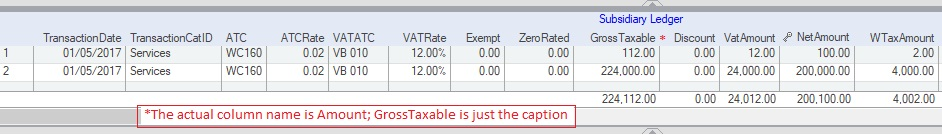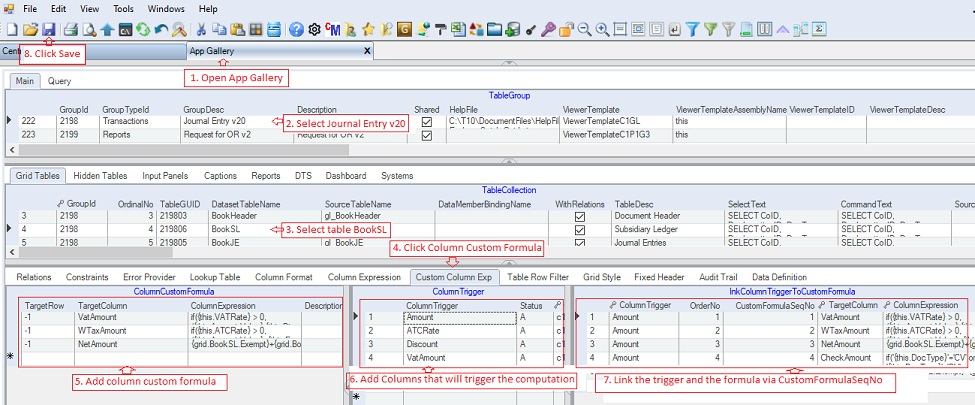Column Custom Formula contains the list of custom expressions which are being evaluated by the parser whenever the user makes changes on the data grid.

There are three data grids in creating custom formula as follows:

1. ColumnCustomFormula that defines the columns and their assocaiated custom formula

2) ColumnTrigger that defines the participating column(s) that will trigger the computation of the formula in #1 above.

3) LnkColumnTriggerToCustomFormula that defines the links between the ColumnCustomFormula and the ColumnTrigger. A change in one column can trigger one or more column custom computations.

In this sample, we will examine the column custom expression of Journal Entry v20 for the table BookSL with grid caption Subsidiary Ledger.The VATAmount, WTaxAmount and NetAmount columns contain column custom expressions to automatically compute their values as seen below.The Column Custom Formula contains the following records for the computation of VATAmout, WTaxAmount and the NetAmount as follows:

 CustomFormula SeqNo* Target Table* Target Row** Target Column**** Column Expression***** Description 1 this -1 VATAmount if({this.VATRate} > 0, ({this.Amount.Value}-{this.Discount.Value})/(1+{this.VATRate})*{this.VATRate},0) Computes the VATAmount 2 this -1 WTaxAmount if({this.ATCRate} > 0, ({this.Amount.Value}+{this.Exempt}+{this.ZeroRated}-{this.Discount.Value}-{this.VatAmount.Value}) * {this.ATCRate.Value}, 0) Computes the WTaxAmount 3 this -1 NetAmount {grid.BookSL.Exempt} + {grid.BookSL.ZeroRated} + {grid.BookSL.Amount} - {grid.BookSL.Discount} - {grid.BookSL.VatAmount} Computes the NetAmount

*CustomFormulaSeqNo serves as a link value to identify the target custom formula to be computed.

**The TargetTable refers to the table where the formula will be applied. The "this" means the current table which is the BookSL. You can specify the name of the target table if it is other than the current table.

***The TargetRow refers to the row where the formula will be posted. The "-1" means the current row. Specify the row number if you are targetting other than the current row which is possible only for fix number of rows.

****Target Column refers to the column where the formula will be appied.

*****Column Expression refers to the formula to be evaluated by the parser. The evaluated value will be posted at the TargetTable->TargetColumn->TargetRow

The above custom column formula will be triggered as follows:

 Column Trigger Link Column Trigger To Custom Formula OrderNo CustomFormulaSeqno Explanations Amount (Gross Taxable) 1 1 Whenever the user change the value in the column Amount (or GrossTaxable), the following will be computed based on the OrderNo as follows: 1. CustomFormulaSeqNo #1 computes the VATAmount 2. CustomFormulaSeqNo #2 computes the WTaxAmount 3. CustomFormulaSeqNo #3 computes the NetAmount 2 2 3 3 ATCRate 1 2 Only CustomFormulaSeqNos #2 and #3 are triggered. We don't need to trigger #1 which is for VATAmount computation since it will not affect the VATAmount when the ATCRate is changed. 2 3 VATAmount, VATRate 1 1 Whenever the user edited the value of VATAmount or the VATRate, we need to trigger CustomFormulaSeqNos #1, #2, #3. We need to recompute the 1=VATAmount, 2=WTaxAmount and 3=NetAmount 2 2 3 3

Step-by-step instructions:

1. Open App Gallery

2. Goto the grid called TableGroup and look for the app named Journal Entry V20

3. Goto TableCollections and select table BookSL where you want to add the custom column formula.

4. Click Column Custom Formula tab

5. Under the Column Custom Formula data grid, add column custom formula. See the topics under Parser category

6. Under the Column Trigger data grid, add the participating columns.

7. Under the lnkColumnTriggerToCustomFomula, link each Column Trigger with the Column Custom Formula via the link key CustomFormulaSeqNo.

8. Click Save.